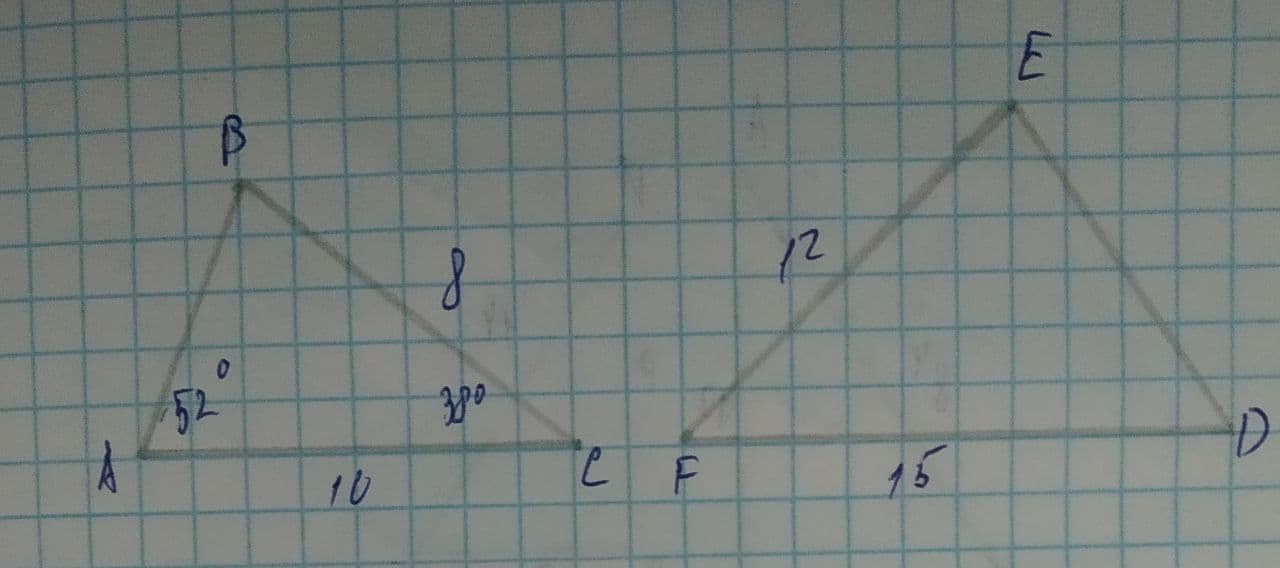# Based on the drawing below, in order for \triangle ABC to be similar to \triangle DEF by SAS similarityTabansi 2021-08-11 Answered
Based on the drawing below, in order for $$\displaystyle\triangle{A}{B}{C}$$ to be similar to $$\displaystyle\triangle{D}{E}{F}$$ by SAS similarity, which of the following needs to be true?A.$$\displaystyle{m}\angle{D}={38}^{{\circ}}$$
B.$$\displaystyle{m}\angle{D}={52}^{{\circ}}$$
C.$$\displaystyle{m}\angle{F}={38}^{{\circ}}$$
D.$$\displaystyle{m}\angle{F}={52}^{{\circ}}$$

• Questions are typically answered in as fast as 30 minutes

### Plainmath recommends

• Get a detailed answer even on the hardest topics.
• Ask an expert for a step-by-step guidance to learn to do it yourself.aprovard
Step 1
Angle C needs to be equal to angle F for these triangles to be similar by SSS.
Step 2
For SAS similarity we need two sides and the angle between them.
We see that $$\displaystyle{\frac{{{B}{C}}}{{{A}{C}}}}={\frac{{{E}{F}}}{{{F}{D}}}}={\frac{{{4}}}{{{5}}}}$$
Hence the $$\displaystyle\angle{B}{C}{A}=\angle{E}{F}{D}\Rightarrow\angle{C}=\angle{F}$$
(The angle BC and AC. and the angle between EF and FD)
$$\displaystyle\Rightarrow\angle{F}=\angle{C}={38}^{{\circ}}$$
$$\displaystyle\Rightarrow{m}\angle{F}={38}^{{\circ}}$$